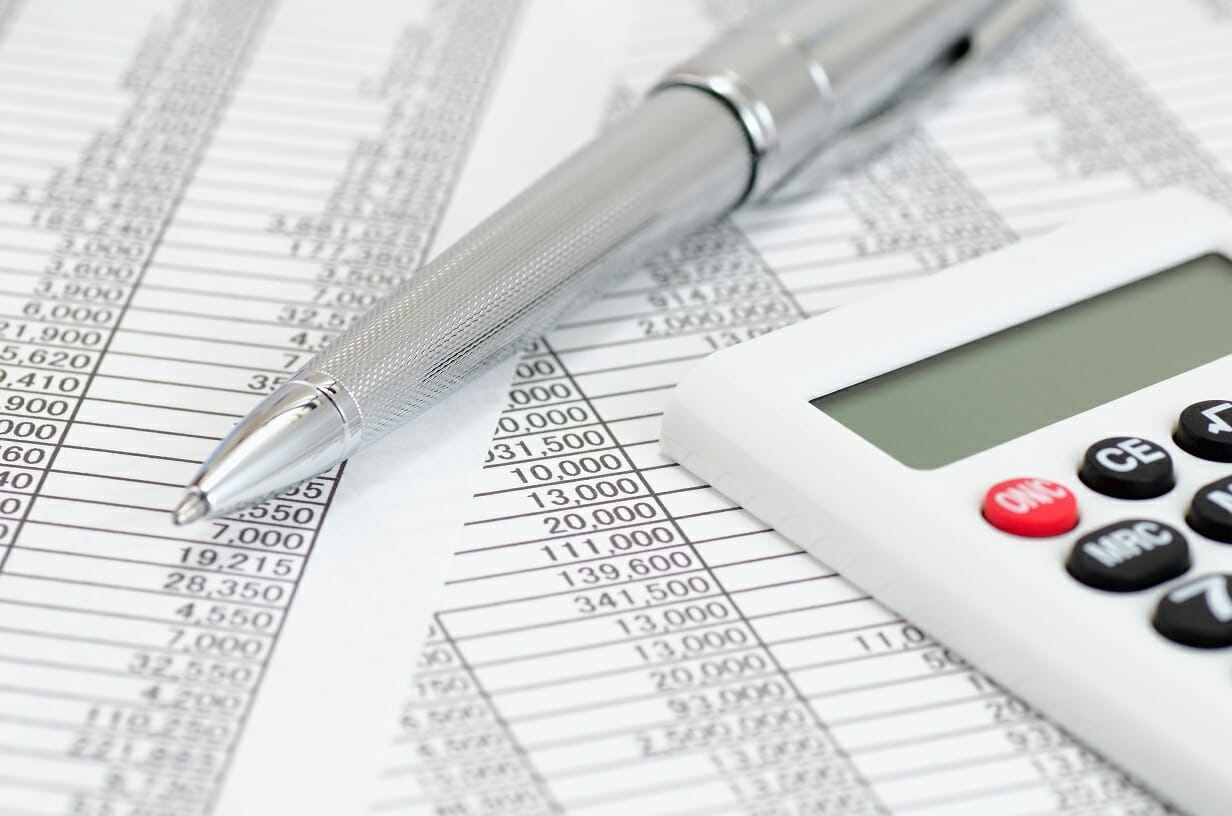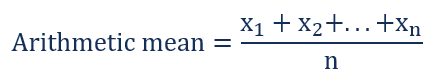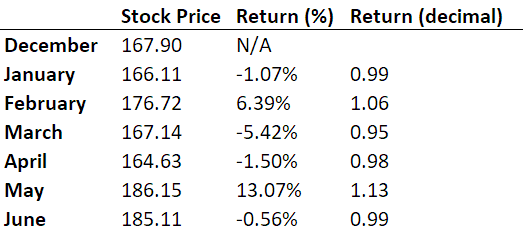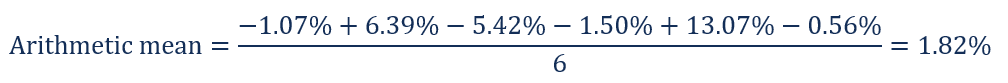# Mean

The average or the most common value in a collection of numbers

# What is Mean?

Mean is an essential concept in mathematics and statistics. In general, the mean is referred to the average or the most common value in a collection of numbers.

In statistics, it is a measure a central tendency of a probability distribution along median and mode. It is also referred to an expected value.

It is a statistical concept also carries a major significance in finance. The concept is used in various financial fields, including but not limited to portfolio management and business valuation.### How to calculate mean?

There are different ways of measuring the central tendency of a set of values, while the average is one of the most popular ones, there are multiple ways to calculate the mean. Here are the two most popular ones:

Arithmetic mean is the total of the sum of all values in a collection of numbers divided by the number of numbers in a collection. It is calculated in the following way:In finance, arithmetic mean may be misleading in the calculations of returns, as it does not consider the effects of volatility and compounding, producing an ‘inflated’ value for the central point of the distribution.

Geometric mean is an nth root of the product of all numbers in a collection. The formula for the geometric mean is:The geometric mean includes the volatility and compounding effects of the returns. Thus, the geometric average provides a more accurate calculation of an average return.

### Arithmetic mean example

Jim wants to find a stock for an investment. He is a big fan of Apple Inc. He knows that the company has strong financials. However, to ensure that this investment will bring him a substantial return he has decided to check how the stock performed in the past. He decides to find the average price of Apple’s share price for the past five months.

He gathered the monthly company’s stock prices from January 2018 to June 2018 and found the monthly returns. The stock prices and returns are summarized in the table below:The formula used for the calculation would be the following:### Geometric mean example

In order to check the obtained result, Jim has decided to calculate the geometric mean return of Apple’s share price. However, it should be calculated not in the percentages but in decimal numbers.

The geometric mean is equal to: